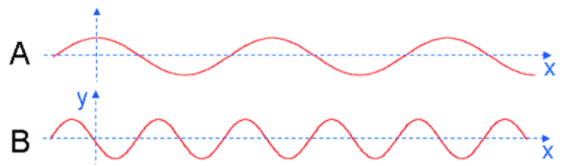# Problem: Waves A and B shown above are propagating in the same medium.1) How do their frequencies compare?a. ωA &lt; ωBb. ωA = ωBc. ωA &gt; ωB2) Briefly explain your answer to the previous question.3) Which one carries the most energy per unit length?a. Ab. Bc. Same4) Briefly explain your answer to the previous question

###### FREE Expert Solution

1.

The relationship between frequency and wavelength is:

$\overline{){\mathbf{f}}{\mathbf{=}}\frac{\mathbf{v}}{\mathbf{\lambda }}}$, where v is the speed of the wave, λ is the wavelength, and f is the frequency.

92% (13 ratings)###### Problem Details

Waves A and B shown above are propagating in the same medium.1) How do their frequencies compare?

a. ω< ωB

b. ωA = ωB

c. ωA > ωB

3) Which one carries the most energy per unit length?

a. A

b. B

c. Same